# Place Value Worksheets

## Teacher Resources made by Other Teachers:

Place Value Math Challenge Workbook - More Difficult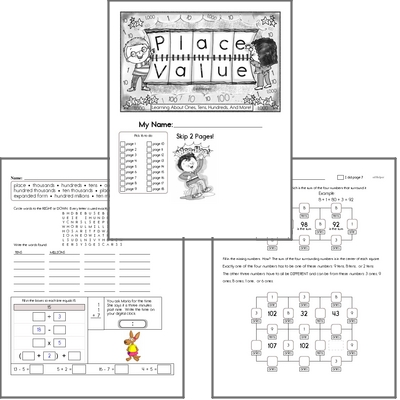Place Value Practice using Fidget Spinners - Fun Workbooks - Kids Love These!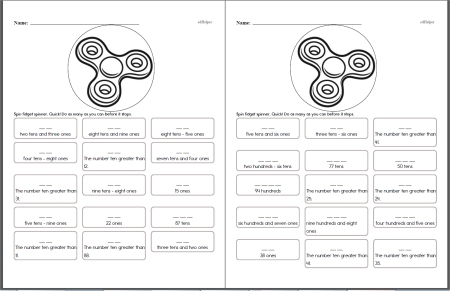Place Value with Fidget Spinner Workbook - All Questions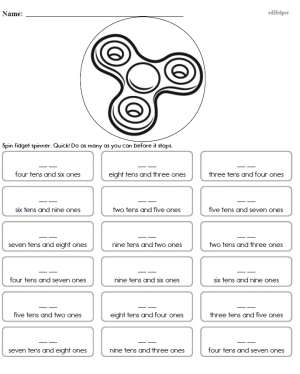Write the number: Such as 5 tens and 3 ones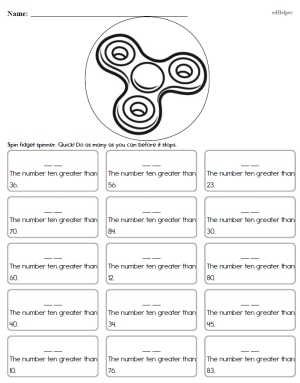Write the number: Such as the number ten greater than 82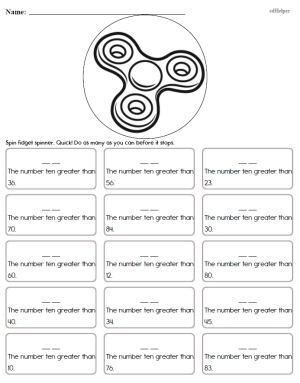Write the number: Such as 15 hundreds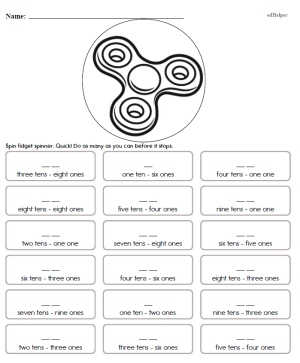Place Value Fluency

Place Value No Prep Book #1
Place Value No Prep Book #1 - Not Boring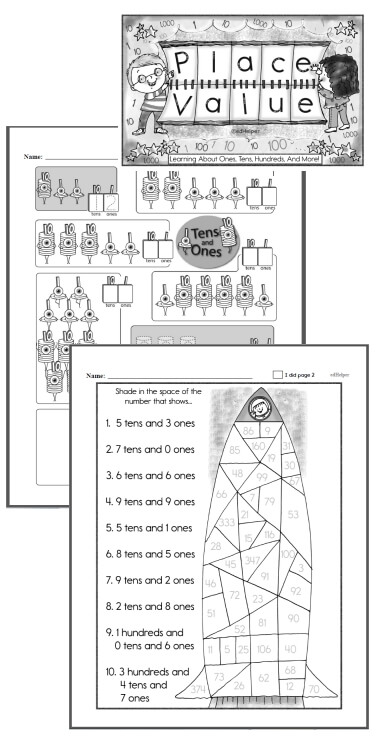Kindergarten - No Prep Place Value Book First Grade - No Prep Place Value Book Second Grade - No Prep Place Value Book

Place Value No Prep Book #2
Place Value No Prep Book #2 - Not Boring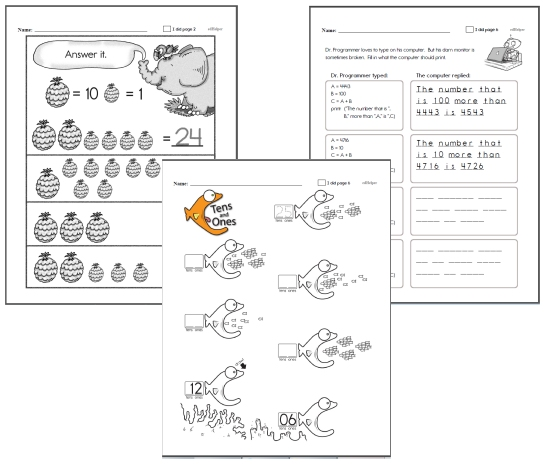Kindergarten - No Prep Place Value Book #2 First Grade - No Prep Place Value Book #2 Second Grade - No Prep Place Value Book #2

I have... Who has... Place Value Activity Game - 2 Digits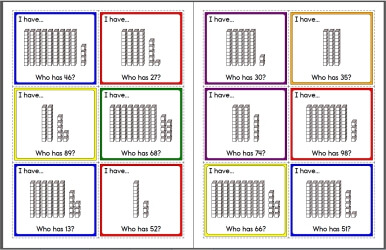Make: I have... Who has... Activity for Classroom

Place Value Math Center - Ones, Tens, and Hundreds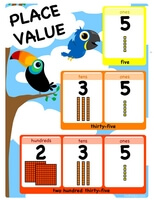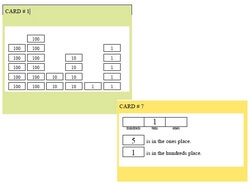Easier: Place value words are given under boxes (first answer given) (Grades 1-3) Easier: Place value words are given under boxes (Grades 1-3) Ones, tens, and hundreds place value math learning center (first answer given) (Grades 1-3) Ones, tens, and hundreds place value math learning center (Grades 1-3)

Place Value Math Center - Ones, Tens, Hundreds, and ThousandsEasier: Place value words are given under boxes (first answer given) (Grades 1-3) Easier: Place value words are given under boxes (Grades 1-3) Ones, tens, hundreds, and thousands place value math learning center (first answer given) (Grades 1-3) Ones, tens, hundreds, and thousands place value math learning center (Grades 1-3)

Place Value Math Center - Ones, Tens, Hundreds, Thousands, and Ten-ThousandsEasier: Place value words are given under boxes (first answer given) (Grades 1-3) Easier: Place value words are given under boxes (Grades 1-3) Ones, tens, hundreds, thousands, and ten-thousands place value math learning center (first answer given) (Grades 1-3) Ones, tens, hundreds, thousands, and ten-thousands place value math learning center (Grades 1-3)

Place Value Charts for Bulletin Board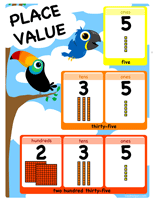Place Value Chart- Ones, Tens, Hundreds (8.5 x 11)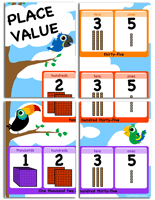Large Place Value Chart - Tens, Hundreds, Thousands (17 x 22)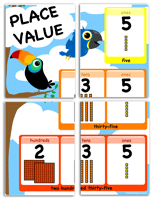Large Place Value Chart - Ones, Tens, Hundreds (17 x 22)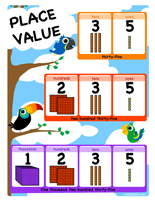Place Value Chart - Ones, Tens, Hundreds, Thousands (8.5 x 11)

Ones, Tens, and Hundreds
Ones, Tens, and Hundreds - Counting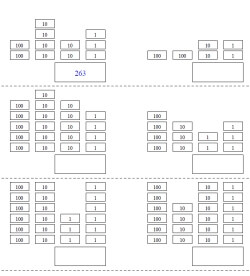Counting (first answer given) Counting

Ones, Tens, and Hundreds - Counting Pictures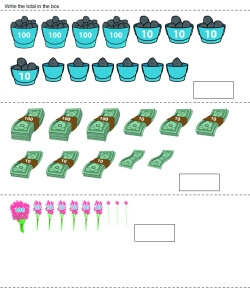Counting

Ones, Tens, and Hundreds - Write the Place Values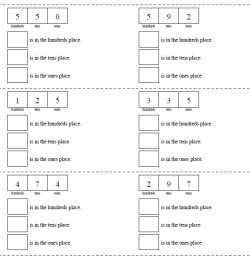Fill in all the places (text hints given for all problems) Fill in all the places (text hints given for first problem) Fill in all the places (no text hints)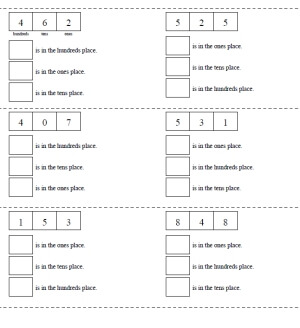Fill in all the places - random order (text hints given for first problem) Fill in all the places - random order (no text hints)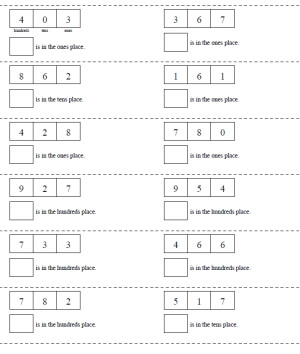Fill in one place (text hints given for first problem) Fill in one place (no text hints)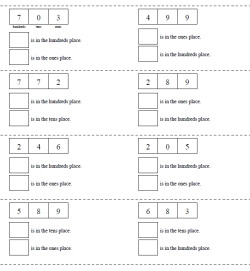Fill in two places (text hints given for first problem) Fill in two places (no text hints)

Ones, Tens, and Hundreds - Write the Number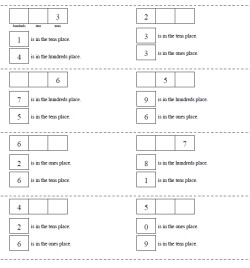Complete the Number: two places given (text hints given for first problem) Complete the Number: two places given (no text hints)

Ones, Tens, and Hundreds - Making a Number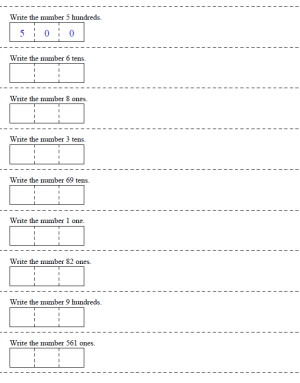Make a number from one place value (first answer given) Make a number from one place value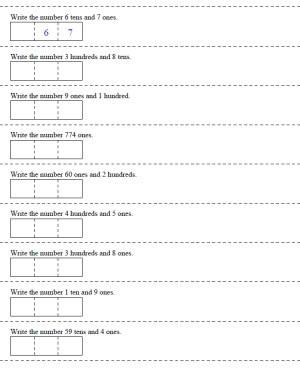Make a number by adding two place values (first answer given) Make a number by adding two place values

Ones, Tens, Hundreds, and Thousands
Ones, Tens, Hundreds, and Thousands - Counting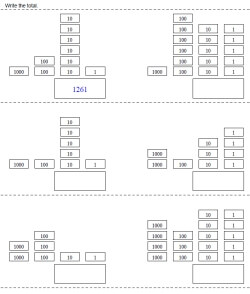Counting (first answer given) Counting

Ones, Tens, Hundreds, and Thousands - Counting Pictures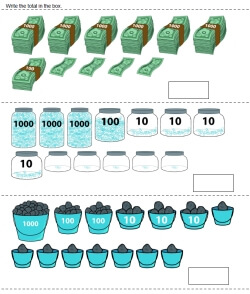Counting

Ones, Tens, Hundreds, and Thousands - Write the Place Values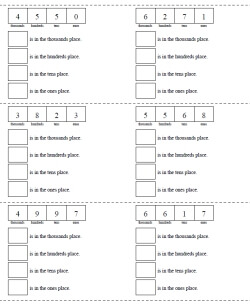Fill in all the places (text hints given for all problems) Fill in all the places (text hints given for first problem) Fill in all the places (no text hints)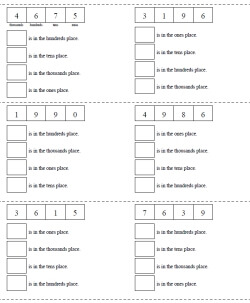Fill in all the places - random order (text hints given for first problem) Fill in all the places - random order (no text hints)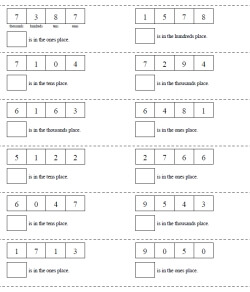Fill in one place (text hints given for first problem) Fill in one place (no text hints)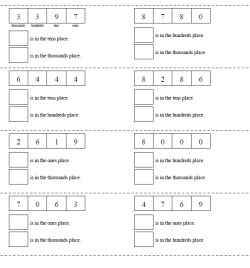Fill in two places (text hints given for first problem) Fill in two places (no text hints)

Ones, Tens, Hundreds, and Thousands - Write the Number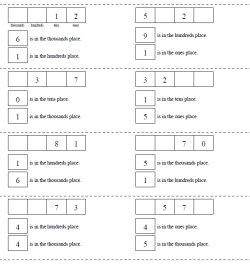Complete the Number: two places given (text hints given for first problem) Complete the Number: two places given (no text hints)

Ones, Tens, Hundreds, and Thousands - Making a Number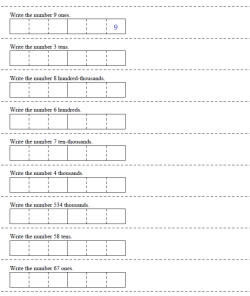Make a number from one place value (first answer given) Make a number from one place value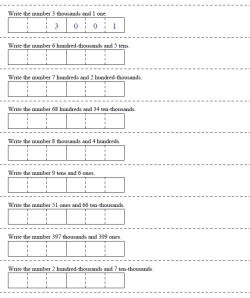Make a number by adding two place values (first answer given) Make a number by adding two place values

Place Value Number Lines
Ones and Tens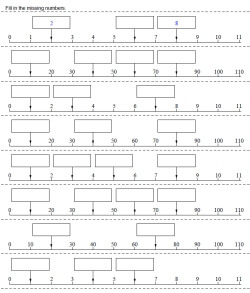Ones and tens number line - all start from 0 (couple answers given) Ones and tens number line - all start from 0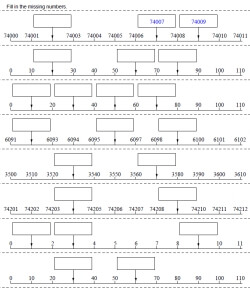Ones and tens number line - half of the problems start from 0 (couple answers given) Ones and tens number line - half of the problems start from 0 Ones and tens number line (couple answers given) Ones and tens number line

Ones, Tens, and Hundreds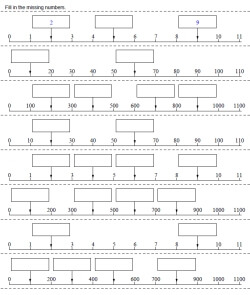Ones, tens, and hundreds number line - all start from 0 (couple answers given) Ones, tens, and hundreds number line - all start from 0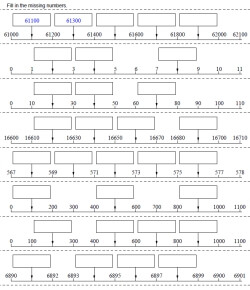Ones, tens, and hundreds number line - half of the problems start from 0 (couple answers given) Ones, tens, and hundreds number line - half of the problems start from 0 Ones, tens, and hundreds number line (couple answers given) Ones, tens, and hundreds number line

Ones, Tens, Hundreds, and Thousands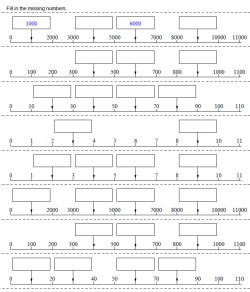Ones, tens, hundreds, and thousands number line - all start from 0 (couple answers given) Ones, tens, hundreds, and thousands number line - all start from 0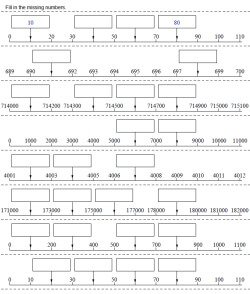Ones, tens, hundreds, and thousands number line - half of the problems start from 0 (couple answers given) Ones, tens, hundreds, and thousands number line - half of the problems start from 0 Ones, tens, hundreds, and thousands number line (couple answers given) Ones, tens, hundreds, and thousands number line

Large Numbers: Up to hundred-thousands
Large Numbers up to hundred-thousands - Write the Place Values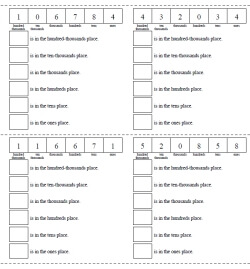Fill in all the places (text hints given for all problems) Fill in all the places (text hints given for first problem) Fill in all the places (no text hints)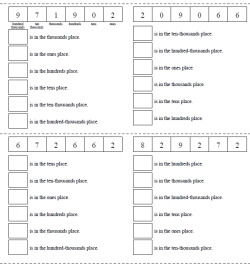Fill in all the places - random order (text hints given for first problem) Fill in all the places - random order (no text hints)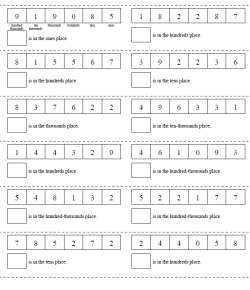Fill in one place (text hints given for first problem) Fill in one place (no text hints)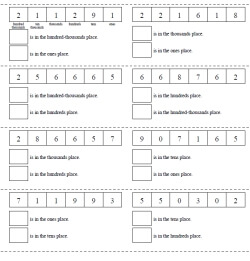Fill in two places (text hints given for first problem) Fill in two places (no text hints)

Large Numbers up to hundred-thousands - Write the Number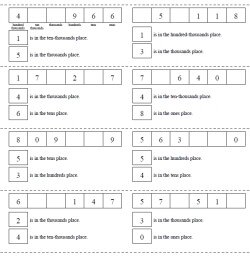Complete the Number: two places given (text hints given for first problem) Complete the Number: two places given (no text hints)

Large Numbers up to hundred-thousands - Making a Number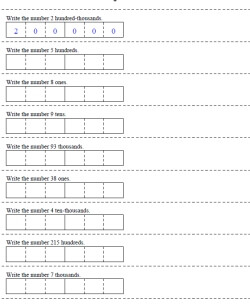Make a number from one place value (first answer given) Make a number from one place value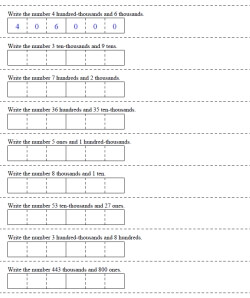Make a number by adding two place values (first answer given) Make a number by adding two place values

Large Numbers: Up to hundred-millions
Large Numbers up to hundred-millions - Write the Place Values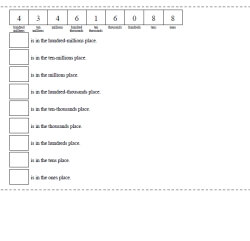Fill in all the places (text hints given for all problems) Fill in all the places (text hints given for first problem) Fill in all the places (no text hints)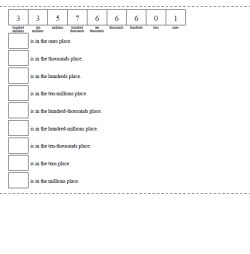Fill in all the places - random order (text hints given for first problem) Fill in all the places - random order (no text hints)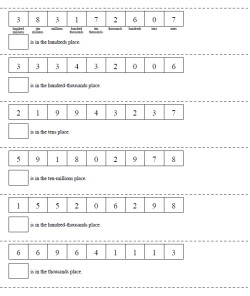Fill in one place (text hints given for first problem) Fill in one place (no text hints)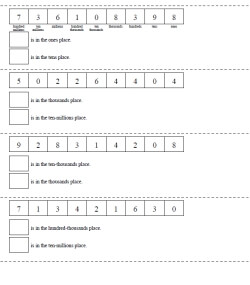Fill in two places (text hints given for first problem) Fill in two places (no text hints)

Large Numbers up to hundred-millions - Write the Number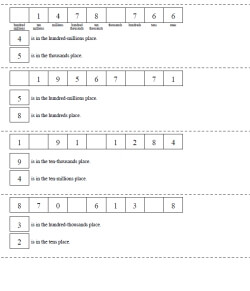Complete the Number: two places given (text hints given for first problem) Complete the Number: two places given (no text hints)

Large Numbers up to hundred-millions - Making a Number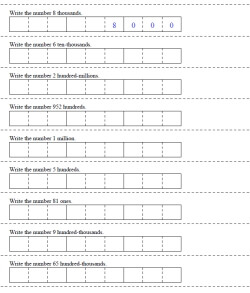Make a number from one place value (first answer given) Make a number from one place value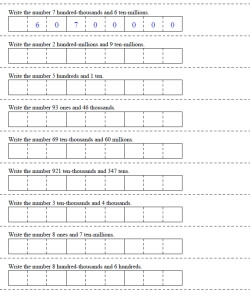Make a number by adding two place values (first answer given) Make a number by adding two place values

Large Numbers: billions and trillions
Large Numbers billions and trillions - Write the Place Values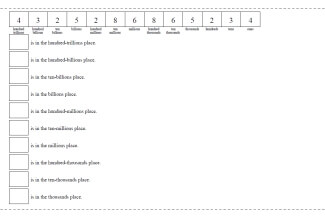Fill in all the places (text hints given for all problems) Fill in all the places (text hints given for first problem) Fill in all the places (no text hints)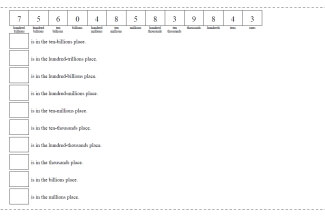Fill in all the places - random order (text hints given for first problem) Fill in all the places - random order (no text hints)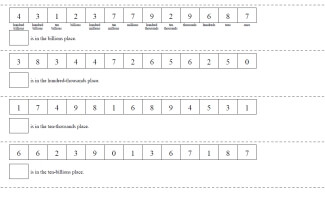Fill in one place (text hints given for first problem) Fill in one place (no text hints)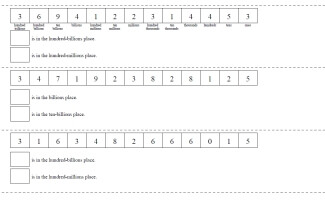Fill in two places (text hints given for first problem) Fill in two places (no text hints)

Large Numbers billions and trillions - Write the Number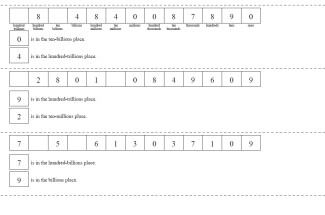Complete the Number: two places given (text hints given for first problem) Complete the Number: two places given (no text hints)

Large Numbers billions and trillions - Making a Number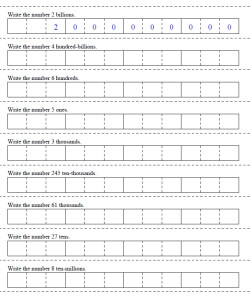Make a number from one place value (first answer given) Make a number from one place value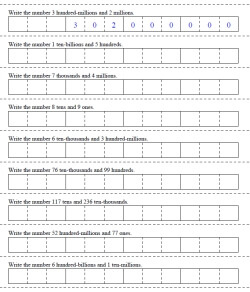Make a number by adding two place values (first answer given) Make a number by adding two place values

Write each number in standard form
Write each number in two other ways
Write the place and the value of the underlined digit
Fill in the missing number
Write each number in standard form (information given is mixed up)
Fill in the missing number (not in order of place value)
Write each number in expanded form using exponents
Write each number in standard form (includes exponents)
Write each number in standard form (decimal place value)
Write each decimal in words (decimal place value)
Write the value of the underlined digit in words (decimal place value)
Write the value of the underlined digit in words (whole numbers and decimal place value)
Place Value Mixed Review

Place Value: Mix: Numbers from Hundreds to Hundred Millions
Write each number in standard form
Write each number in two other ways
Write the place and the value of the underlined digit
Fill in the missing number
Write each number in standard form (information given is mixed up)
Fill in the missing number (information given is mixed up)
Ones, Tens, and Hundreds Mixed Review
Write each number in expanded form using exponents
Write each number in standard form (includes exponents)
Review with exponents

Hundreds Chart

Place Value: Ones, Tens, and Hundreds
Write each number in standard form
Write each number in two other ways
Write the place and the value of the underlined digit
Fill in the missing number
Write each number in standard form (information given is mixed up)
Fill in the missing number (information given is mixed up)
Ones, Tens, and Hundreds Mixed Review
Write each number in expanded form using exponents
Write each number in standard form (includes exponents)
Review with exponents

Tens and Ones
Tens and Ones (Graphic)
Tens and Ones
Tens and Ones Number Comparison (Largest Number)
Tens and Ones Number Comparison (Smallest Number)
Tens and Ones Puzzle

Place Value Math Center - Ones, Tens, and HundredsEasier: Place value words are given under boxes (first answer given) (Grades 1-3) Easier: Place value words are given under boxes (Grades 1-3) Ones, tens, and hundreds place value math learning center (first answer given) (Grades 1-3) Ones, tens, and hundreds place value math learning center (Grades 1-3)

Place Value Math Center - Ones, Tens, Hundreds, and ThousandsEasier: Place value words are given under boxes (first answer given) (Grades 1-3) Easier: Place value words are given under boxes (Grades 1-3) Ones, tens, hundreds, and thousands place value math learning center (first answer given) (Grades 1-3) Ones, tens, hundreds, and thousands place value math learning center (Grades 1-3)

Place Value Math Center - Ones, Tens, Hundreds, Thousands, and Ten-ThousandsEasier: Place value words are given under boxes (first answer given) (Grades 1-3) Easier: Place value words are given under boxes (Grades 1-3) Ones, tens, hundreds, thousands, and ten-thousands place value math learning center (first answer given) (Grades 1-3) Ones, tens, hundreds, thousands, and ten-thousands place value math learning center (Grades 1-3)

Hundreds, Tens, and Ones
Tens and Ones
Hundreds, Tens and Ones
Hundreds, Tens, and Ones Puzzle

Place Value: Through Thousands
Write each number in standard form
Write each number in two other ways
Write the place and the value of the underlined digit
Fill in the missing number
Write each number in standard form (information given is mixed up)
Fill in the missing number (information given is mixed up)
Ones, Tens, and Hundreds Mixed Review
Write each number in expanded form using exponents
Write each number in standard form (includes exponents)
Review with exponents

Thousands, Hundreds, Tens, and Ones
Hundreds, Tens and Ones
Thousands, Hundreds, Tens and Ones
Thousands, Hundreds, Tens and Ones
Thousands, Hundreds, Tens, and Ones Puzzle

Rounding
Rounding Tens, Hundreds, and Thousands
Rounding Puzzle

Place Value: Through Ten Thousands
Write each number in standard form
Write each number in two other ways
Write the place and the value of the underlined digit
Fill in the missing number
Write each number in standard form (information given is mixed up)
Fill in the missing number (information given is mixed up)
Ones, Tens, and Hundreds Mixed Review
Write each number in expanded form using exponents
Write each number in standard form (includes exponents)
Review with exponents

Place Value: Through Thousands, Ten Thousands, and Hundred Thousands
Write each number in standard form
Write each number in two other ways
Write the place and the value of the underlined digit
Fill in the missing number
Write each number in standard form (information given is mixed up)
Fill in the missing number (information given is mixed up)
Ones, Tens, and Hundreds Mixed Review
Write each number in expanded form using exponents
Write each number in standard form (includes exponents)
Review with exponents

Place Value: Through Millions, Ten Millions, and Hundred Millions
Write each number in standard form
Write each number in two other ways
Write the place and the value of the underlined digit
Fill in the missing number
Write each number in standard form (information given is mixed up)
Fill in the missing number (information given is mixed up)
Ones, Tens, and Hundreds Mixed Review
Write each number in expanded form using exponents
Write each number in standard form (includes exponents)
Review with exponents

Leveled Books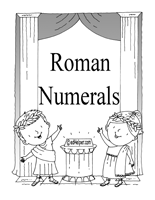Roman Numerals

Math Place Value Logic Printables
Students: match grade levels and id numbers
What is each person's number?
Number of pennies

Decimals Place Value: Tenths and Hundredths
Write each number in standard form (decimal place value)
Write each decimal in words (decimal place value)
Write the value of the underlined digit in words (decimal place value)
Write the value of the underlined digit in words (whole numbers and decimal place value)
Decimals: Tenths and Hundredths Review

Decimals Place Value: Through Thousandths
Write each number in standard form (decimal place value)
Write each decimal in words (decimal place value)
Write the value of the underlined digit in words (decimal place value)
Write the value of the underlined digit in words (whole numbers and decimal place value)
Decimals: Through Thousandths Review

Decimals Place Value: Through Ten-Thousandths
Write each number in standard form (decimal place value)
Write each decimal in words (decimal place value)
Write the value of the underlined digit in words (decimal place value)
Write the value of the underlined digit in words (whole numbers and decimal place value)
Decimals: Through Ten-Thousandths Review

Roman Numeral Worksheets
Translate from Roman Numerals (Numbers 1-32)
Translate to Roman Numerals (Numbers 1-32)
Translate to and from Roman Numerals (Numbers 1-32)
Translate from Roman Numerals (Numbers 1950-2050)
Translate to Roman Numerals (Numbers 1950-2050)
Translate to and from Roman Numerals (Numbers 1950-2050)
Translate from Roman Numerals (Numbers 1-100)
Translate to Roman Numerals (Numbers 1-100)
Translate to and from Roman Numerals (Numbers 1-100)
Translate from Roman Numerals (Numbers 1-3000)
Translate to Roman Numerals (Numbers 1-3000)
Translate to and from Roman Numerals (Numbers 1-3000)
Subtraction (Numbers 1-40)
Subtraction (Numbers 1-90)
Review Crossword Puzzle - Mix of Everything (Numbers 1-50)
Review Crossword Puzzle - Mix of Everything (Numbers 1-150)
Review Crossword Puzzle - Mix of Everything (Numbers 1-1000)

How to Write Chinese Numbers
Writing Chinese Characters to Represent Numbers (Rules)
Shows how to write the numbers 1 to 7
Shows how to write the numbers 8 to 100 million

Practice Writing Chinese Characters
Writing the Chinese Character for 0
Writing the Chinese Character for 1
Writing the Chinese Character for 2
Writing the Chinese Character for 3
Writing the Chinese Character for 4
Writing the Chinese Character for 5
Writing the Chinese Character for 6
Writing the Chinese Character for 7
Writing the Chinese Character for 8
Writing the Chinese Character for 9
Writing the Chinese Character for 10
Writing the Chinese Character for 100
Writing the Chinese Character for 1,000
Writing the Chinese Character for 10,000

Chinese Numbers: Complete The Table
Numbers 10 to 99
Numbers 10 to 99 - Expanded Format: (9 x 10)
Numbers 10 to 99 - Expanded Format: (9 x 101)
Numbers 10 to 999
Numbers 10 to 999 - Expanded Format: (9 x 100)
Numbers 10 to 999 - Expanded Format: (9 x 102)
Numbers 10 to 9999
Numbers 10 to 9999 - Expanded Format: (9 x 1000)
Numbers 10 to 9999 - Expanded Format: (9 x 103)
Numbers 10 to 99999
Numbers 10 to 99999 - Expanded Format: (9 x 10000)
Numbers 10 to 99999 - Expanded Format: (9 x 105)

Crossword Puzzles
Chinese Numbers Crossword (2 Digits)
Chinese Numbers Crossword (2-3 Digits)
Chinese Numbers Crossword (2-4 Digits)
Chinese Numbers Crossword (2-5 Digits)

Word Search
Chinese Numbers Word Search (2 Digits)
Chinese Numbers Word Search (2-3 Digits)
Chinese Numbers Word Search (2-4 Digits)
Chinese Numbers Word Search (2-5 Digits)

Test Prep - Numeration (Includes Place Value Questions)
from the test prep section, requires registration